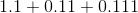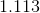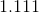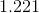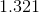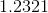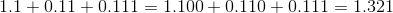## Example Questions

### Example Question #1 : Problem Solving

Jenny is getting ready for school. She bought school supplies on a tax-free day. She bought notebooks for $1.25 each and crayons for$0.90 each. Jenny bought two more notebooks than crayons. She paid with a ten dollar bill and got \$1.05 in change. How many notebooks did she buy?

2

4

5

1

3

5

Explanation:

Define variables as x = number of crayons and x + 2 = number of notebooks

To find out how much she spent, we subtract her change from what she paid with: 10.00 – 1.05 = 8.95

Then we need to solve the problem cost of notebooks plus the cost of crayons equals the total cost.

1.25(x + 2) + 0.90x = 8.95 and solving for x gives 3 crayons and 5 notebooks

### Example Question #1 : Other Decimals

Simplify the following: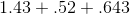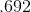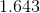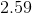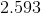Explanation:

To add decimals, first add zeros to the necessary terms so they all have the same number of places to the right of the decimal point.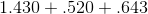Now, temporarily drop the decimal and add the numbers as if they were whole numbers.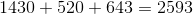Finally, add the decimal place back in, in the same place you removed it from. In this case, we originally modified our numbers so they all had 3 numbers to the right of the decimal, so we will add the decimal back to the exact same place. Thus, our answer is:### Example Question #177 : Decimals

Add: